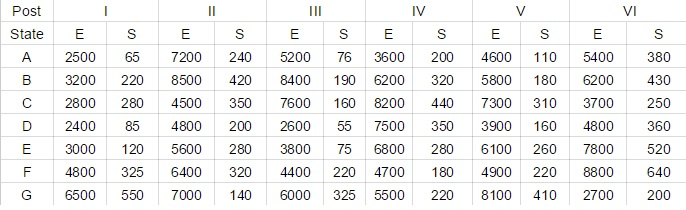## IBPS Quant Test 20

Instructions

Study the following table carefully and answer the question which follows
Number of candidates found Eligible and the
Number of candidates short listed for Interview for a recent Recruitment process for six posts from different statesQuestion 1

From state B which post had the highest percentage of candidates short listed ?

Question 2

What is the average number of candidates (approximately) found eligible for post III from all states ?

Question 3

What is the overall percentage (rounded off to one digit after decimal) of candidates short listed over the total number of candidates eligible for post I from all the states together ?

Question 4

What is the ratio of the total number of candidates short listed for all the posts together from state E to that from state G ?

Question 5

The total number of candidates found eligible for post I from all states together is approximately what per cent of the total of candidates found eligible for post VI from all states together ?